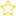Resources for teaching statistics: Key Resources, Teacher Preparation

We revise key ideas from the “Random Error” videos on the question, “How wrong could I be?” We then introduce thoughts about margins of error and confidence intervals as a way forward, but run up against a seemingly insurmountable problem.

After you’ve watched this video, you should be able to answer these questions:

• What is the most reliable way we know of obtaining data about populations without misleading biases? Why is this method not perfect?
• What happens whenever we use data from a sample to estimate a population quantity?
• What do we do about that?
• If you have an estimate of a population quantity and know its margin of error, within what limits should the true value lie?
• Why does estimating the extent of sampling error in a practical application seem like an impossible problem?

If you couldn’t answer a question, you might find it helpful to look at the Illustrated transcript.

## Rate this resource:(No ratings yet)